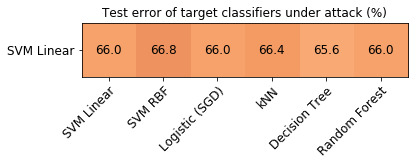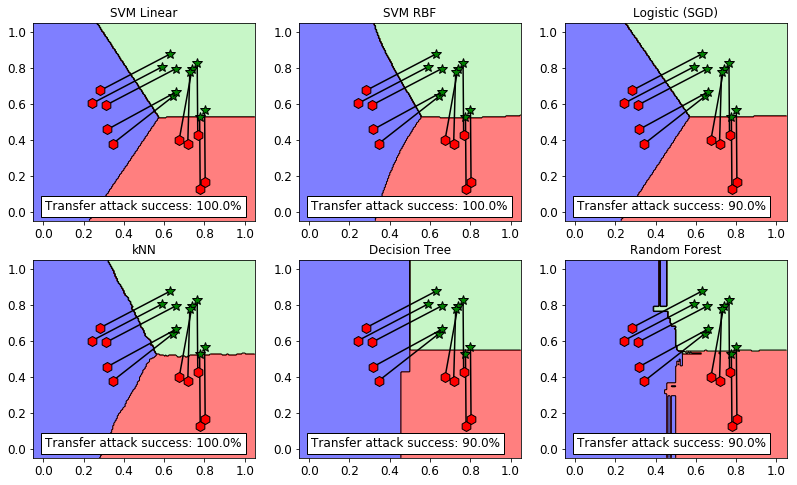# 2.2. Transferability of Evasion Attacks¶

Transferability captures the ability of an attack against a machine-learning model to be effective against a different, potentially unknown, model.

In this tutorial we are going to test if an evasion attack generated against a Support Vector Machine (SVM), the surrogate classifier, will transfer to other classifiers, the targets, or not.

[demontis19-usenix] Demontis, A., Melis, M., Pintor, M., Jagielski, M., Biggio, B., Oprea, A., Nita-Rotaru, C. and Roli, F., 2019. Why Do Adversarial Attacks Transfer? Explaining Transferability of Evasion and Poisoning Attacks. In 28th Usenix Security Symposium, Santa Clara, California, USA.

We will first create and train the surrogate and different target classifiers, evaluating their performance in the standard scenario, i.e. not under attack. The surrogate and the target classifiers will be trained on different training sets.

The following part partially replicates the procedure from the first tutorial.

:

random_state = 999

n_features = 2  # Number of features
n_samples = 2250  # Number of samples
centers = [[-2, 0], [2, -2], [2, 2]]  # Centers of the clusters
cluster_std = 0.8  # Standard deviation of the clusters

dataset = CDLRandomBlobs(n_features=n_features,
centers=centers,
cluster_std=cluster_std,
n_samples=n_samples,

n_tr = 1000  # Number of training set samples
n_ts = 250  # Number of test set samples

# Split in training and test.
from secml.data.splitter import CTrainTestSplit
splitter = CTrainTestSplit(
train_size=2 * n_tr, test_size=n_ts, random_state=random_state)
tr, ts = splitter.split(dataset)

# Normalize the data
from secml.ml.features import CNormalizerMinMax
nmz = CNormalizerMinMax()
tr.X = nmz.fit_transform(tr.X)
ts.X = nmz.transform(ts.X)

# Generate 2 training datasets for surrogate and target classifiers
tr1 = tr[:n_tr, :]  # Source classifier training set
tr2 = tr[:n_tr, :]  # Target classifier training set

from collections import namedtuple
CLF = namedtuple('CLF', 'clf_name clf xval_parameters')

from secml.ml.classifiers.multiclass import CClassifierMulticlassOVA
# Binary classifiers
from secml.ml.classifiers import CClassifierSVM, CClassifierSGD
# Natively-multiclass classifiers
from secml.ml.classifiers import CClassifierKNN, CClassifierDecisionTree, CClassifierRandomForest

# Let's create a 3-Fold data splitter
from secml.data.splitter import CDataSplitterKFold
xval_splitter = CDataSplitterKFold(num_folds=3, random_state=random_state)

# Metric to use for training and performance evaluation
from secml.ml.peval.metrics import CMetricAccuracy
metric = CMetricAccuracy()

surr_clf = CLF(
clf_name='SVM Linear',
clf=CClassifierMulticlassOVA(CClassifierSVM, kernel='linear'),
xval_parameters={'C': [1e-2, 0.1, 1]})

print("Estimating the best training parameters of the surrogate classifier...")
best_params = surr_clf.clf.estimate_parameters(
dataset=tr1,
parameters=surr_clf.xval_parameters,
splitter=xval_splitter,
metric=metric,
perf_evaluator='xval'
)

print("The best training parameters of the surrogate classifier are: ",
[(k, best_params[k]) for k in sorted(best_params)])

surr_clf.clf.fit(tr1)

y_pred = surr_clf.clf.predict(ts.X)

acc = metric.performance_score(y_true=ts.Y, y_pred=y_pred)

print("Accuracy of the surrogate classifier on test set: {:.2%}".format(acc))

print("\nTraining the target classifiers...")

target_clf_list = [
CLF(
clf_name='SVM Linear',
clf=CClassifierMulticlassOVA(CClassifierSVM, kernel='linear'),
xval_parameters={'C': [1e-2, 0.1, 1]}),
CLF(clf_name='SVM RBF',
clf=CClassifierMulticlassOVA(CClassifierSVM, kernel='rbf'),
xval_parameters={'C': [1e-2, 0.1, 1],  'kernel.gamma': [1, 10, 100]}),
CLF(clf_name='Logistic (SGD)',
clf=CClassifierMulticlassOVA(
CClassifierSGD, regularizer='l2', loss='log',
random_state=random_state),
xval_parameters={'alpha': [1e-6, 1e-5, 1e-4]}),
CLF(clf_name='kNN',
clf=CClassifierKNN(),
xval_parameters={'n_neighbors': [30, 40, 50]}),
CLF(clf_name='Decision Tree',
clf=CClassifierDecisionTree(),
xval_parameters={'max_depth': [1, 3, 5]}),
CLF(clf_name='Random Forest',
clf=CClassifierRandomForest(random_state=random_state),
xval_parameters={'n_estimators': [20, 30, 40]}),
]

for i, test_case in enumerate(target_clf_list):

clf = test_case.clf
xval_params = test_case.xval_parameters

print("\nEstimating the best training parameters of {:} ..."
"".format(test_case.clf_name))

best_params = clf.estimate_parameters(
dataset=tr2, parameters=xval_params, splitter=xval_splitter,
metric='accuracy', perf_evaluator='xval')

print("The best parameters for '{:}' are: ".format(test_case.clf_name),
[(k, best_params[k]) for k in sorted(best_params)])

print("Training of {:} ...".format(test_case.clf_name))
clf.fit(tr2)

# Predictions on test set and performance evaluation
y_pred = clf.predict(ts.X)
acc = metric.performance_score(y_true=ts.Y, y_pred=y_pred)

print("Classifier: {:}\tAccuracy: {:.2%}".format(test_case.clf_name, acc))

Estimating the best training parameters of the surrogate classifier...
The best training parameters of the surrogate classifier are:  [('C', 0.1)]
Accuracy of the surrogate classifier on test set: 99.60%

Training the target classifiers...

Estimating the best training parameters of SVM Linear ...
The best parameters for 'SVM Linear' are:  [('C', 0.1)]
Training of SVM Linear ...
Classifier: SVM Linear  Accuracy: 99.60%

Estimating the best training parameters of SVM RBF ...
The best parameters for 'SVM RBF' are:  [('C', 0.1), ('kernel.gamma', 10)]
Training of SVM RBF ...
Classifier: SVM RBF     Accuracy: 99.20%

Estimating the best training parameters of Logistic (SGD) ...
The best parameters for 'Logistic (SGD)' are:  [('alpha', 1e-05)]
Training of Logistic (SGD) ...
Classifier: Logistic (SGD)      Accuracy: 99.60%

Estimating the best training parameters of kNN ...
The best parameters for 'kNN' are:  [('n_neighbors', 40)]
Training of kNN ...
Classifier: kNN Accuracy: 99.20%

Estimating the best training parameters of Decision Tree ...
The best parameters for 'Decision Tree' are:  [('max_depth', 3)]
Training of Decision Tree ...
Classifier: Decision Tree       Accuracy: 99.20%

Estimating the best training parameters of Random Forest ...
The best parameters for 'Random Forest' are:  [('n_estimators', 30)]
Training of Random Forest ...
Classifier: Random Forest       Accuracy: 98.40%


## 2.2.1. Generation of the Adversarial Examples¶

As done in the evasion tutorial, we now craft the adversarial examples using the gradient-based maximum-confidence algorithm for generating evasion attacks, implemented by the CAttackEvasionPGDLS class (e-pgd-ls).

This time, we are going to generate an error-specific attack by setting y_target to one of the classes of the dataset. In this way, we enforce the solver to perturb the points so that the classifier will classify them with the y_true label.

Please note that the attack on multiple samples may take a while (up to a few minutes) depending on the machine the script is run on.

:

noise_type = 'l2'  # Type of perturbation 'l1' or 'l2'
dmax = 0.4  # Maximum perturbation
lb, ub = 0, 1  # Bounds of the attack space. Can be set to None for unbounded
y_target = 2  # error-specific attack. None for error-generic

# Should be chosen depending on the optimization problem
solver_params = {
'eta': 1e-1,
'eta_min': 0.1,
'eta_max': None,
'max_iter': 100,
'eps': 1e-4
}

pgd_ls_attack = CAttackEvasionPGDLS(
classifier=surr_clf.clf,
surrogate_classifier=surr_clf.clf,
surrogate_data=tr1,
distance=noise_type,
dmax=dmax,
lb=lb, ub=ub,
solver_params=solver_params,
y_target=y_target)

# Run the evasion attack on x0
print("Attack started...")
y_pred, scores, adv_ds, f_obj = pgd_ls_attack.run(ts.X, ts.Y, double_init=False)
print("Attack complete!")

Attack started...
Attack complete!


## 2.2.2. Analysis of Transferability¶

Let’s now test if the previously generated examples transfer to other models.

Initally, we test the performance of each target classifier on the adversarial examples. Later, we plot few of the samples on a 2D plane.

:

# Metric to use for testing transferability
from secml.ml.peval.metrics import CMetricTestError
metric = CMetricTestError()

trans_error = []
transfer_rate = 0.0
for target_clf in target_clf_list:

print("\nTesting transferability of {:}".format(target_clf.clf_name))

origin_error = metric.performance_score(
y_true=ts.Y, y_pred=target_clf.clf.predict(ts.X))

print("Test error (no attack): {:.2%}".format(origin_error))

trans_error_clf = metric.performance_score(

trans_error.append(trans_error_clf)
transfer_rate += trans_error_clf

# Computing the transfer rate
transfer_rate /= len(target_clf_list)

from secml.array import CArray
trans_acc = CArray(trans_error) * 100  # Show results in percentage

from secml.figure import CFigure
# Only required for visualization in notebooks
%matplotlib inline

fig = CFigure(height=1)
a = fig.sp.imshow(trans_acc.reshape((1, 6)),
cmap='Oranges', interpolation='nearest',
alpha=.65, vmin=60, vmax=70)

fig.sp.xticks(CArray.arange((len(target_clf_list))))
fig.sp.xticklabels([c.clf_name for c in target_clf_list],
rotation=45, ha="right", rotation_mode="anchor")
fig.sp.yticks()
fig.sp.yticklabels([surr_clf.clf_name])

for i in range(len(target_clf_list)):
fig.sp.text(i, 0, trans_acc[i].round(2).item(), va='center', ha='center')

fig.sp.title("Test error of target classifiers under attack (%)")

fig.show()

print("\nAverage transfer rate: {:.2%}".format(transfer_rate))


Testing transferability of SVM Linear
Test error (no attack): 0.40%

Testing transferability of SVM RBF
Test error (no attack): 0.80%

Testing transferability of Logistic (SGD)
Test error (no attack): 0.40%

Testing transferability of kNN
Test error (no attack): 0.80%

Testing transferability of Decision Tree
Test error (no attack): 0.80%

Testing transferability of Random Forest
Test error (no attack): 1.60%Average transfer rate: 66.13%


We can observe how the accuracy of the target classifiers on the adversarial point generated against the surrogate classifier is extremely low, which highlights how the machine-learning models are vulnerable to transfer attacks.

:

from secml.figure import CFigure
from secml.array import CArray
from math import ceil
fig = CFigure(width=4.5 * len(target_clf_list) / 2,
height=4 * 2, markersize=10)

for clf_idx in range(len(target_clf_list)):
clf = target_clf_list[clf_idx].clf

fig.subplot(2, int(ceil(len(target_clf_list) / 2)), clf_idx + 1)
fig.sp.title(target_clf_list[clf_idx].clf_name)

fig.sp.plot_decision_regions(clf, n_grid_points=200)
fig.sp.grid(grid_on=False)

s_idx = ts.Y.find(ts.Y != y_target)

for pt in s_idx[:10]:  # Plot the translation of multiple adversarial samples
pt_segment = CArray.append(ts.X[pt, :], adv_ds.X[pt, :], axis=0)
fig.sp.plot_path(pt_segment)

acc = metric.performance_score(These 2D plot clearly visualize the vulnerability of the target classifiers. The adversarial examples (green stars) which are inside the y_target = 2 green decision region, are successfully transfered.